# Matrix summation method

(diff) ← Older revision | Latest revision (diff) | Newer revision → (diff)
Jump to: navigation, search

One of the methods for summing series and sequences using an infinite matrix. Employing an infinite matrix,a given sequenceis transformed into the sequence: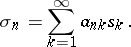If the series on the right-hand side converges for alland if the sequence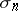has a limitfor: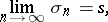then the sequenceis said to be summable by the method determined by the matrix, or simply summable by the matrix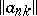, and the numberis referred to as its limit in the sense of this summation method. If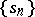is regarded as the sequence of partial sums of a series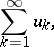(1)

then this series is said to be summable to the sumby the matrix.

A matrix summation method for series can be also defined directly by transforming the series (1) into a sequence: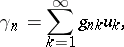(2)

where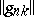is a given matrix. In this case the series (1) is said to be summable to the sumif, for all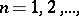the series on the right-hand side in (2) converges and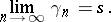Less often used are matrix summation methods defined by a transformation of a series (1) into a series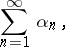(3)

where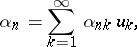or by a transformation of a sequenceinto a series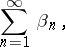(4)

where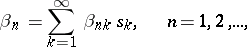which use matrices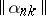and, respectively. In these cases the series (1) with the partial sums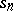is summable to the sumif the series (3) converges toor, respectively, if the series (4) converges to.

The matrix of a summation method all entries of which are non-negative is called a positive matrix. Among the matrix summation methods one finds, for example, the Voronoi summation method, the Cesàro summation methods, the Euler summation method, the Riesz summation method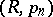, the Hausdorff summation method, and others (see also Summation methods).

How to Cite This Entry:
Matrix summation method. Encyclopedia of Mathematics. URL: http://encyclopediaofmath.org/index.php?title=Matrix_summation_method&oldid=12057
This article was adapted from an original article by I.I. Volkov (originator), which appeared in Encyclopedia of Mathematics - ISBN 1402006098. See original article